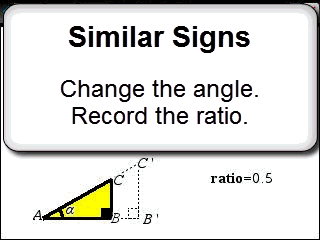Activities

•• Subject Area

• Mathematics: Algebra and Pattern: Algebra and Pattern
• Mathematics: Measurement: Measurement
• Mathematics: Geometry and Space: Geometry and Space
• Standard: National Curriculum 7-10: Measurement and Geometry: Shape
• Standard: National Curriculum 7-10: Measurement and Geometry: Geometric reasoning

• AuthorMiddle

60 Minutes

• Device
•TI-Nspire™ CX
• TI-Nspire™ CAS
• Software

TI-Nspire™
TI-Nspire™ CAS

3.2

Similar SignsActivity Overview

Students investigate the ratio between the opposite side and hypotenuse of a right angled triangle as the triangle is dilated. Once students identify the outcome they explore changes to the angle and estimate ratios for specific angles.

Objectives

Use similarity to investigate the constancy of the sine ratio for a given angle in right-angled triangles (ACMMG223)

Vocabulary

• Ratio
• Similarity
• Opposite (side)